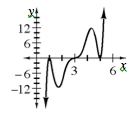### Home > CCA2 > Chapter Ch12 > Lesson 12.1.3 > Problem12-55

12-55.

Quickly sketch each of the following functions.

1. $f(x) = (x - 1)^2(x - 3)^3(x - 5)^2$2. $g(x) = -(x - 1)^2(x - 3)^3(x - 5)^2$

How is this different from the function in part (a)?
What transformation is created by a negative sign?

3. Express $g(x)$ in terms of $f(x)$.

Look at your graphs from parts (a) and (b).
What do they tell you about the relationship between the two functions?

$g(x) = -f(x)$